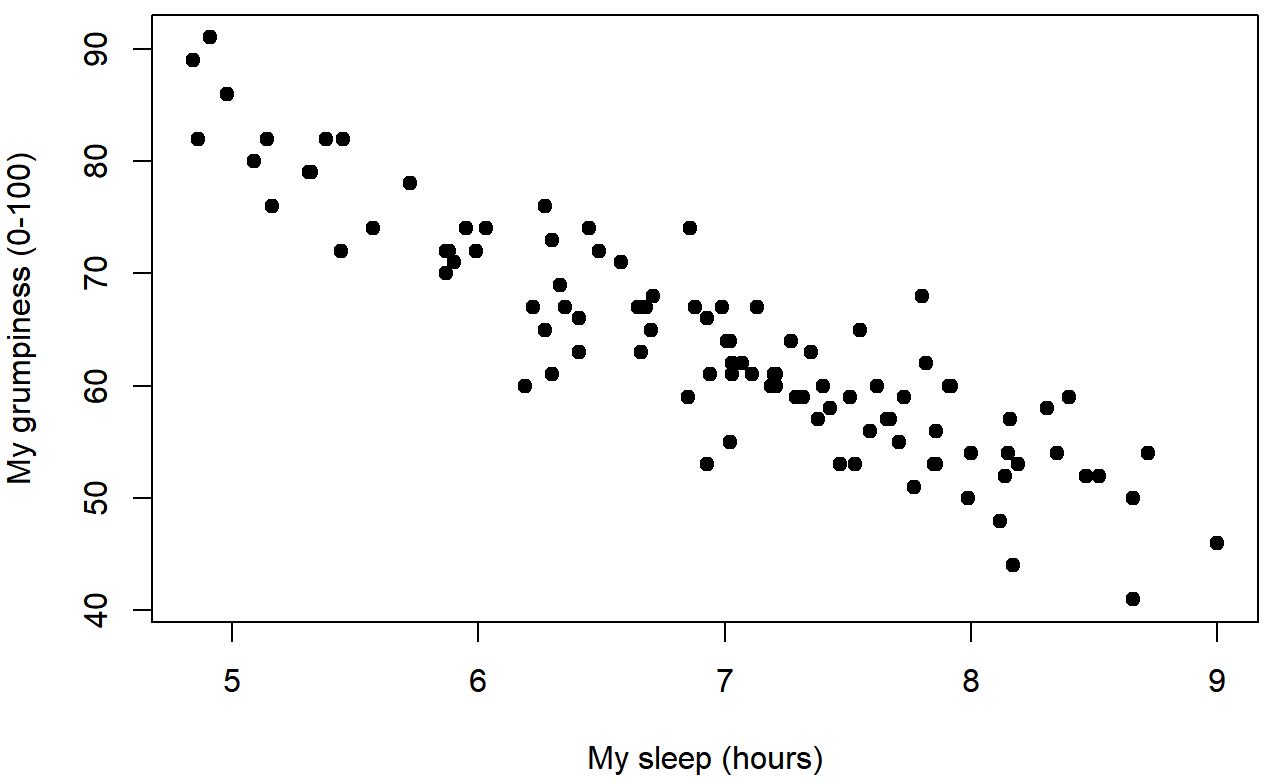# 15.1: What Is a Linear Regression Model?Figure 15.1: Scatterplot showing grumpiness as a function of hours slept.Figure 15.2: Panel a shows the sleep-grumpiness scatterplot from above with the best fitting regression line drawn over the top. Not surprisingly, the line goes through the middle of the data.Figure 15.3: In contrast, this plot shows the same data, but with a very poor choice of regression line drawn over the top.

Since the basic ideas in regression are closely tied to correlation, we’ll return to the parenthood.Rdata file that we were using to illustrate how correlations work. Recall that, in this data set, we were trying to find out why Dan is so very grumpy all the time, and our working hypothesis was that I’m not getting enough sleep. We drew some scatterplots to help us examine the relationship between the amount of sleep I get, and my grumpiness the following day. The actual scatterplot that we draw is the one shown in Figure 15.1, and as we saw previously this corresponds to a correlation of r=−.90, but what we find ourselves secretly imagining is something that looks closer to Figure 15.2. That is, we mentally draw a straight line through the middle of the data. In statistics, this line that we’re drawing is called a regression line. Notice that – since we’re not idiots – the regression line goes through the middle of the data. We don’t find ourselves imagining anything like the rather silly plot shown in Figure 15.3.

This is not highly surprising: the line that I’ve drawn in Figure 15.3 doesn’t “fit” the data very well, so it doesn’t make a lot of sense to propose it as a way of summarising the data, right? This is a very simple observation to make, but it turns out to be very powerful when we start trying to wrap just a little bit of maths around it. To do so, let’s start with a refresher of some high school maths. The formula for a straight line is usually written like this:

y=mx+c

Or, at least, that’s what it was when I went to high school all those years ago. The two variables are x and y, and we have two coefficients, m and c. The coefficient m represents the slope of the line, and the coefficient c represents the y-intercept of the line. Digging further back into our decaying memories of high school (sorry, for some of us high school was a long time ago), we remember that the intercept is interpreted as “the value of y that you get when x=0”. Similarly, a slope of m means that if you increase the x-value by 1 unit, then the y-value goes up by m units; a negative slope means that the y-value would go down rather than up. Ah yes, it’s all coming back to me now.

Now that we’ve remembered that, it should come as no surprise to discover that we use the exact same formula to describe a regression line. If Y is the outcome variable (the DV) and X is the predictor variable (the IV), then the formula that describes our regression is written like this:

$$\ \hat{Y_i} = b_1X_i + b_0$$

Hm. Looks like the same formula, but there’s some extra frilly bits in this version. Let’s make sure we understand them. Firstly, notice that I’ve written Xi and Yi rather than just plain old X and Y. This is because we want to remember that we’re dealing with actual data. In this equation, Xi is the value of predictor variable for the ith observation (i.e., the number of hours of sleep that I got on day i of my little study), and Yi is the corresponding value of the outcome variable (i.e., my grumpiness on that day). And although I haven’t said so explicitly in the equation, what we’re assuming is that this formula works for all observations in the data set (i.e., for all i). Secondly, notice that I wrote $$\ \hat{Y_i}$$ and not Yi. This is because we want to make the distinction between the actual data Yi, and the estimate $$\ \hat{Y_i}$$ (i.e., the prediction that our regression line is making). Thirdly, I changed the letters used to describe the coefficients from m and c to b1 and b0. That’s just the way that statisticians like to refer to the coefficients in a regression model. I’ve no idea why they chose b, but that’s what they did. In any case b0 always refers to the intercept term, and b1 refers to the slope.

Excellent, excellent. Next, I can’t help but notice that – regardless of whether we’re talking about the good regression line or the bad one – the data don’t fall perfectly on the line. Or, to say it another way, the data Yi are not identical to the predictions of the regression model $$\ \hat{Y_i}$$. Since statisticians love to attach letters, names and numbers to everything, let’s refer to the difference between the model prediction and that actual data point as a residual, and we’ll refer to it as ϵi.214 Written using mathematics, the residuals are defined as:

$$\ \epsilon_i = Y_i - \hat{Y_i}$$

which in turn means that we can write down the complete linear regression model as:

$$\ Y_i = b_1X_i + b_0 + \epsilon_i$$